# Colleges with the highest SAT scores in Pennsylvania

Top 10 colleges in Pennsylvania with the highest SAT scores
Looking for the colleges with the highest SAT scores in Pennsylvania? Well you're in luck! We've compiled a national college database and have created a list of the top 10 universities with the highest SAT scores in Pennsylvania. These are the schools whose applicants had the highest average SAT scores in Pennsylvania. And, since these tests are meant to determine academic prowess, they are arguably the schools with the most academically proficient students. You could even say these are the best colleges in Pennsylvania. We also include each college's ACT scores and acceptance rate so that you can see where you would have the easiest or hardest time getting in. Read on to find out more.

## Carnegie Mellon University SAT scores

The average SAT score for Carnegie Mellon University is 1510.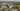The average SAT score of 1510 breaks down into:

• SAT math: 780

The average ACT score for Carnegie Mellon University is 34 and their acceptance rate is 15.4%.

## University of Pennsylvania SAT scores

The average SAT score for University of Pennsylvania is 1500.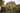The average SAT score of 1500 breaks down into:

• SAT math: 770

The average ACT score for University of Pennsylvania is 34 and their acceptance rate is 8.4%.

## Swarthmore College SAT scores

The average SAT score for Swarthmore College is 1470.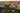The average SAT score of 1470 breaks down into:

• SAT math: 750

The average ACT score for Swarthmore College is 32 and their acceptance rate is 9.5%.

## Haverford College SAT scores

The average SAT score for Haverford College is 1450.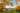The average SAT score of 1450 breaks down into:

• SAT math: 740

The average ACT score for Haverford College is 33 and their acceptance rate is 18.8%.

## Bryn Mawr College SAT scores

The average SAT score for Bryn Mawr College is 1410.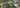The average SAT score of 1410 breaks down into:

• SAT math: 710

The average ACT score for Bryn Mawr College is 31 and their acceptance rate is 34.1%.

## Villanova University SAT scores

The average SAT score for Villanova University is 1395.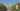The average SAT score of 1395 breaks down into:

• SAT math: 715

The average ACT score for Villanova University is 33 and their acceptance rate is 28.2%.

## Lehigh University SAT scores

The average SAT score for Lehigh University is 1365.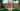The average SAT score of 1365 breaks down into:

• SAT math: 710

The average ACT score for Lehigh University is 31 and their acceptance rate is 32.1%.

## Franklin & Marshall College SAT scores

The average SAT score for Franklin & Marshall College is 1360.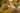The average SAT score of 1360 breaks down into:

• SAT math: 700

The average ACT score for Franklin & Marshall College is 30 and their acceptance rate is 35.3%.

## University of Pittsburgh SAT scores

The average SAT score for University of Pittsburgh is 1350.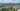The average SAT score of 1350 breaks down into:

• SAT math: 685

The average ACT score for University of Pittsburgh is 31 and their acceptance rate is 56.7%.

## Gettysburg College SAT scores

The average SAT score for Gettysburg College is 1340.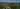The average SAT score of 1340 breaks down into:

• SAT math: 670